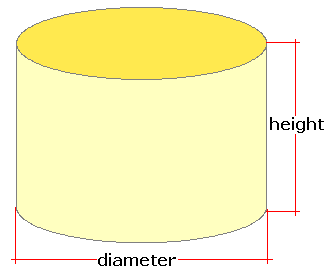Spike's Calculators

# Number of Bushels in a Cylinder Shaped Bin

Estimate the number of bushels in a round grain bin. Calculation includes the volume of the bin in cubic feet and litres. The United States uses the Winchester bushel (1.244456 ft²), and in Canada, they use the Avery bushel ( 1.284349 ft²).##### Volume Formula
```V = Π (D/2)²H;
where V is the volume of the bin
Π = 3.14159
D = the diameter of the bin
H = the height of the bin
```
##### Conversions
```one bushel (bu) US = 1.24445601851852 cubic feet (ft³)
one bushel (bu) Imperial = 1.28434922588714 cubic feet (ft³)
one cubic foot (ft³) = 28.316846592 litres (L)```

### Number of Bushels-Grain Bin-Round

Diameter ft
Bin Height ft

#### Results:

 Bin Volume in Cubic Feet ft³ Litres L Imperial Bushels bu Imp US Bushels lbs

#### Calculator

1. diameter of the bin in feet
2. height of the bin in feet

#### Results

1. bin volume in cubic feet
2. bin volume in litres
3. number of US (Winchester) bushels
4. number of Imperial (Avery) bushels